×

Search anything:

# Squeeze and Excitation (SE) Network

#### Machine Learning (ML)Get this book -> Problems on Array: For Interviews and Competitive Programming

This article describes what are Convolutional Neural Network and What are Squeeze and Excitation blocks.

1. Introduction
2. What are Convolutional Neural Networks?
3. What are Squeeze-and-Excitation Networks and it’s Architecture?
3.1 Squeeze: Global Information Embedding
4. Implementation
5. Summary

# Introduction:

Squeeze-and-Excitation Networks, bring in a building block for Convolutional Neural Networks (CNN) that increase channel interdependencies at nearly no cost of computation.Basically Squeeze-and-excitation blocks, are an architectural element that may be placed into a convolutional neural network to boost performance while only increasing the overall number of parameters by a minimal amount.

Before moving on to What are Squeeze-and-Excitation blocks, lets first understand what are Convolutional Neural Networks.

# What are Convolutional Neural Networks? (CNN):

A convolutional neural network (CNN) is an artificial neural network that is especially built to analyse pixel data and is used in image recognition and processing.

CNNs are image processing, artificial intelligence (AI) systems that employ deep learning to do both generative and descriptive tasks, frequently utilising machine vision that includes image and video recognition, as well as recommender systems and natural language processing (NLP).

They are divided into three sorts of layers:

• Convolutional layer
• Pooling layer
• Fully-connected (FC) layer

## 1. Convolutional Layer:

The convolutional layer is the most important component of a CNN because it is where the majority of the computation takes place. It requires input data, a filter, and a feature map. The first layer to extract features from an input image is the convolutional layer.

## 2. Pooling Layer:

The Pooling layer, like the Convolutional Layer, is responsible for shrinking the Convolved Feature's spatial size. Through dimensionality reduction, the computational power required to process the data is reduced. It's also beneficial for extracting rotational and positional invariant dominating features, which helps keep the model's training process running smoothly.

Pooling can be divided into two categories:

Max pooling: The filter picks the pixel with the highest value to transmit to the output array as it advances across the input. In comparison to average pooling, this strategy is employed more frequently.

Average pooling: The filter calculates the average value inside the receptive field as it passes across the input and sends it to the output array.

# 3. Fully-connected (FC) layer

The full-connected layer's name is self-explanatory. In partly linked layers, the pixel values of the input picture are not directly connected to the output layer. On the other hand, each node in the output layer, links directly to a node in the preceding layer in the fully-connected layer.

This layer's purpose is to execute classification tasks using characteristics derived from preceding layers and their respective filters. While convolutional and pooling layers typically utilise ReLu functions to categorise inputs, FC layers typically use a softmax activation function to get a probability from 0 to 1.

Now since we have an understanding of what convolutional neural networks are, let's get into Squeeze-and-Excitation blocks.

# What are Squeeze-and-Excitation Networks and it’s Architecture:

Let's first understand the main idea behind it,

The main idea behind Squeeze-and-Excitation Networks:

Is to add parameters to every convolutional block's channel so that the network could modify the weight of every feature map adaptively.

In simple words, the squeeze-and-excitation block improves the neural networks' ability to map channel dependence while also providing access to global information. As a result, they are better able to adjust the filter outputs, resulting in improved performance.

Now, Let’s understand Squeeze and Excitation step:

• For the “squeeze” step, what we do is we use global average pooling to aggregate feature maps across their spatial dimensions H x W to produce channel descriptor.

• And for the “excitation” step, what we do is we apply fully connected layers to the output of the “squeeze” step to produce a collection of per-channel weights (“activations”) which are applied to the feature maps in order to generate the final output of the SE block.

The below image shows the SE Block.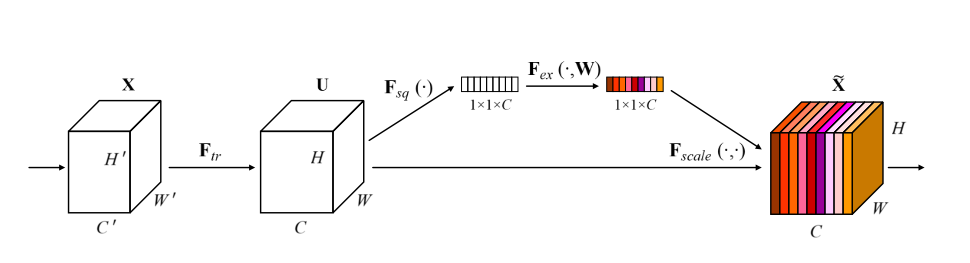Let’s get into more detail:

## 3.1 Squeeze: Global Information Embedding

The SE block's "squeeze" stage compresses global spatial information into a channel description. To provide channel-wise statistics, the squeeze stage consists of global average pooling across the spatial dimensions H x W. Here's an excerpt that includes the squeeze step's explanation and equation: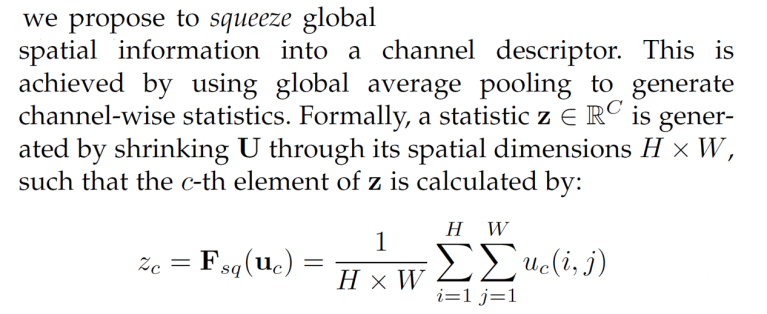The squeeze process, which converts each separate H x W feature map u c into a scalar channel descriptor z c, is depicted in the diagram below: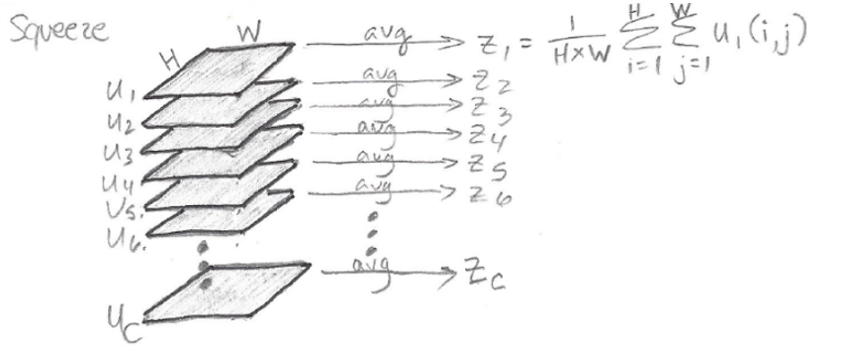The scalars [z 1, z 2,..., z C] combine to generate a C-length vector z, which will be utilised in the excitation stage.

The excitation procedure is designed to capture channel-specific dependencies completely. The excitation operation converts the squeeze step's output (the vector z) into a vector of activations s, which is then used to rescale the feature maps.

The vector s is developed using two completely-connected layers that reduces the representation to size C/r: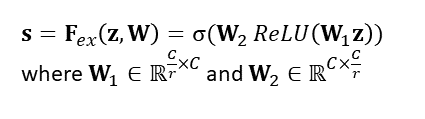The "reduction ratio" is the name given to the hyperparameter r. The intermediate representation shrinks as r is larger. The purpose of shrinking the representation to C/r and then extending it back to C is to (a) reduce model complexity and (b) improve generalisation.

The activations in s are estimated from the squeeze output z. Below is the diagram: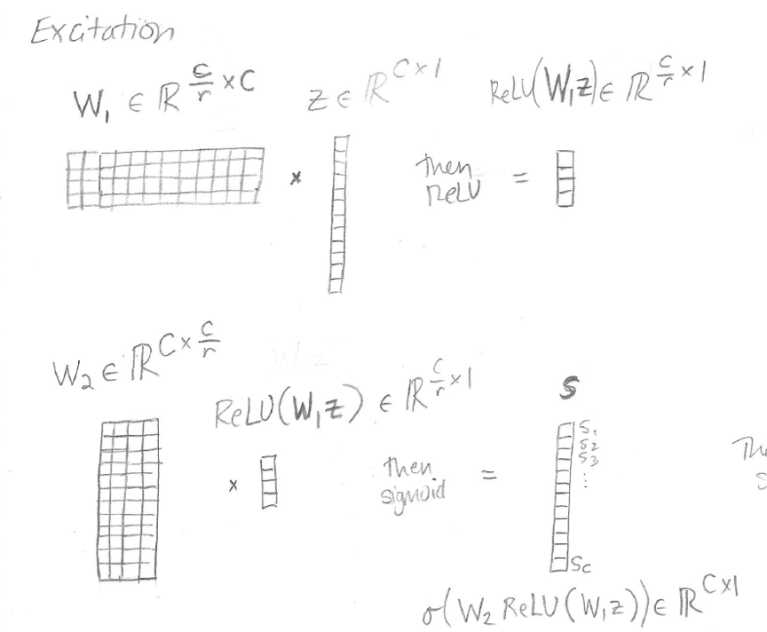# 4. Implementation:

Any CNN architecture can benefit from a squeeze-and-excitation block. Lets see how one can use an SE block in a ResNet by a diagram: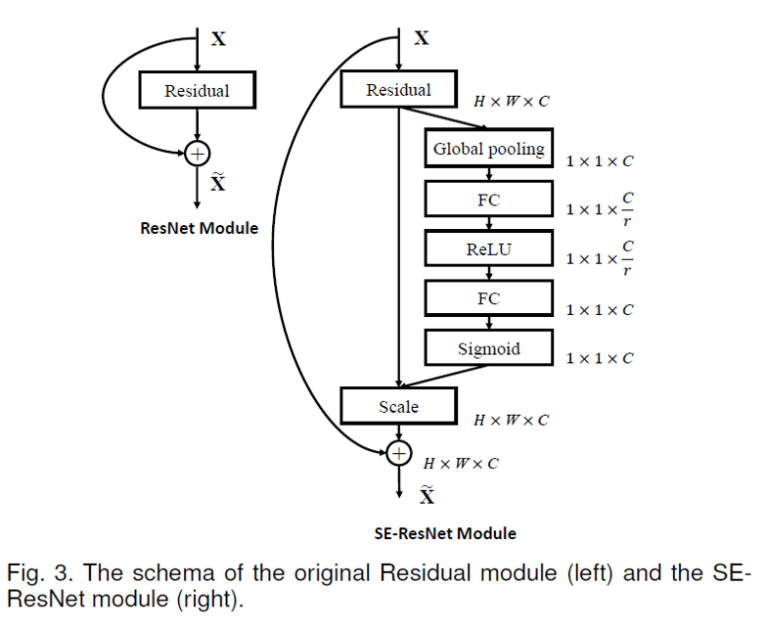We could also conclude that by adding SE-blocks to ResNet-50, you can get almost the same level of accuracy as ResNet-101. This is outstanding for a model that only requires half the processing resources.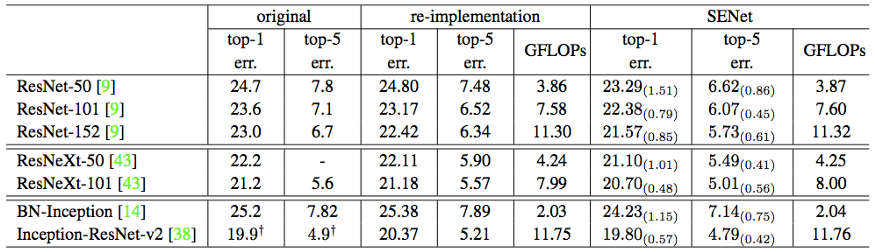The above image depicsts How SENets improve existing architectures

Let’s Summarize everything:

# 5. Summary:

• Squeeze-and-excitation blocks explicitly describe channel interactions and interdependencies, as well as a form of self-attention on channel.

• Squeeze-and-excitation blocks re-calibrate feature maps by utilising a "squeeze" operation of global average pooling followed by a "excitation" operation using two completely linked layers.

• Squeeze-and-excitation blocks may be integrated into any CNN architecture/ structure and have a low computational cost.

• Squeeze-and-excitation blocks have been shown to increase classification and object identification performance.

With this article at OpenGenus, you must have the complete idea of Squeeze and Excitation (SE) Networks.

#### Aman Shrivastav

Aman Shrivastav is a Machine Learning Developer, Intern at OpenGenus. He is, currently pursuing B. Tech in Computer Science at Mumbai University.

Squeeze and Excitation (SE) Network